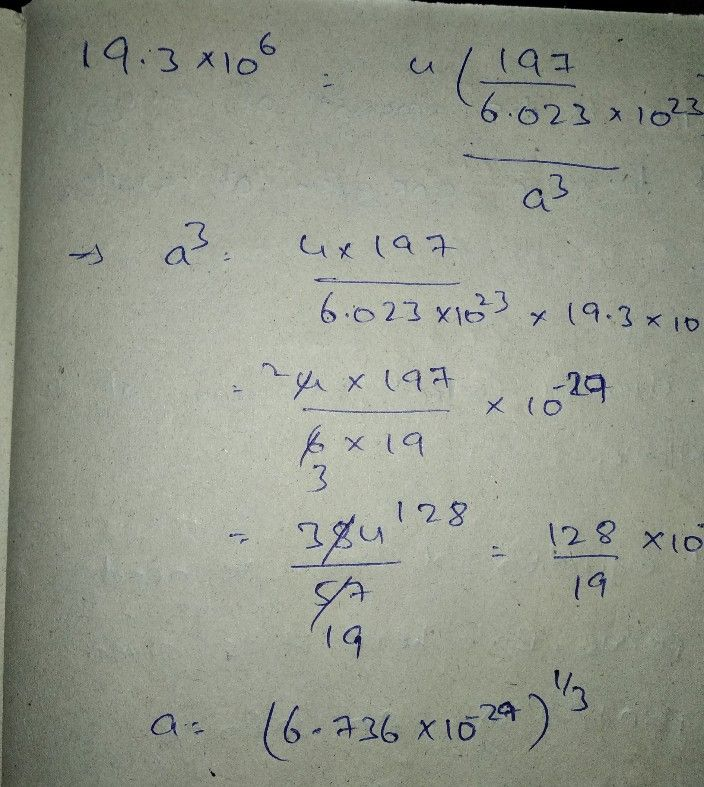Symbol
ProblemFind the first and second derivatives of $f\left(x\right)atx=1.5$ if $x$ $1.5$ $2.0$ $25$ $f\left(x\right)$ $3.375$ $7.000$ $13.625$ $\dfrac {3.0} {24.000}$ $3.5$ $4.0$ $38.875$ $59.000$
Calculus
SolutionQanda teacher - shivaramakif uncleared please do ask again